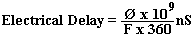# Measuring Characteristic Impedance and Effective Dielectric Constant of PCB tracks using a Vector Network Analyser page 2

First the delay is calculated using the total phase shift of the measured 20 cm of the SHORT track at the test frequency.Formula 2

Where Ø = Total Phase Shift of track (degrees)

f = Frequency ( MHz)

Dr Eric Bogatin first calculates equivalent velocity, but velocity factor is a more useful parameter in the laboratory.Formula 3

Where c = Speed of light = 30 (cm/nS)

l = mechanical length (cm)Formula 4

Where Vf = Velocity FactorFormula 5

Where εeff = Effective Dielectric Constant

Removing Connectors From Results

One of the major problems with measuring a short electrical length is that the impedance of the connectors and terminations cause a large error because they are a large proportion of the measured circuit. This problem is avoided in this method in two ways.

i) The input connectors are three identical SMA connectors mounted tight against the PCB. This means that any variations in length for this part of the circuit is very small in relation to the overall measurement track length which is 22 cm long. The VNA is calibrated with RBW 300 Hz and Average = 16 in order to improve accuracy and then connected to the SHORT 2 cm PCB track. The phase length of the 2 cm track is removed from the test by performing a port extension with the VNA. This means that the measurement plane is 2 cm along the track. When the VNA is now connected to a measurement track, it measures the last 20 cm of the 22 cm track and ignores the first 2 cm and the connector.

ii) Two separate tracks are used for the OPEN and SHORT measurements. This removes the problem of using a SHORT or OPEN termination which have a short length with an impedance (probably 50Ω). The method also avoids the difficulty of physically shorting an open track without changing it's physical length.

Procedure

The VNA was calibrated and a port extension performed as per Method on page one. The OPEN track was connected first and the S11 trace stored in DISPLAY. The VNA was then transferred to the SHORT track and measurements were made at + 900 with each complete revolution of phase recorded. The data for each point was calculated and a graph constructed which is shown in Figure 1 below.It can be seen the there is a ripple in Zo that is not present in the other three characteristics. This suggests that the measured amplitude has a ripple, but the measured phase does not. It can also be seen that the first measurement varies considerably from the others.

1  2  3  >  Page

Technical Article Index